CS 1010 Programming Methodology http www comp nus

• Slides: 50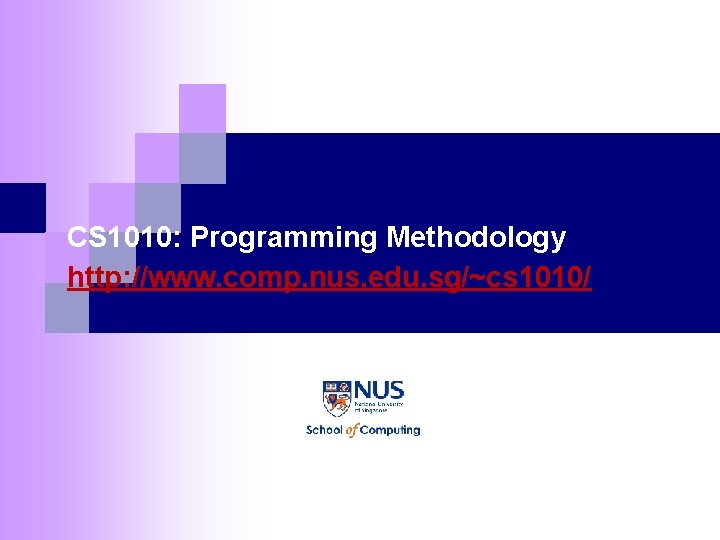CS 1010: Programming Methodology http: //www. comp. nus. edu. sg/~cs 1010/Week 10: Searching and Sorting Objectives: § Understand the basic searching algorithms and § § sorting algorithms Introduce the concept of complexity analysis (informally) Implement the searching and sorting algorithms using arrays. Reference: § Chapter 6 Numeric Arrays § Lesson 6. 6 Bubble Sort, Exchange Maximum Sort CS 1010 (AY 2012/3 Semester 1) Week 10 - 2Week 10: Outline (1/2) 1. Week 9 Exercise #3 Arrow Program 2. Overall Introduction 3. Introduction to Searching 4. Linear Search § Demo #1 § Performance 5. Exercise #1: Compatible Teammate 6. Binary Search CS 1010 (AY 2012/3 Semester 1) Week 10 - 3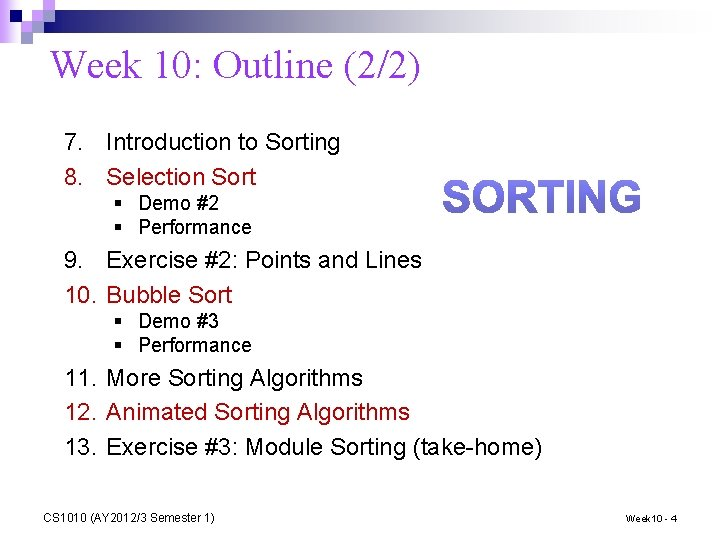Week 10: Outline (2/2) 7. Introduction to Sorting 8. Selection Sort § Demo #2 § Performance 9. Exercise #2: Points and Lines 10. Bubble Sort § Demo #3 § Performance 11. More Sorting Algorithms 12. Animated Sorting Algorithms 13. Exercise #3: Module Sorting (take-home) CS 1010 (AY 2012/3 Semester 1) Week 10 - 4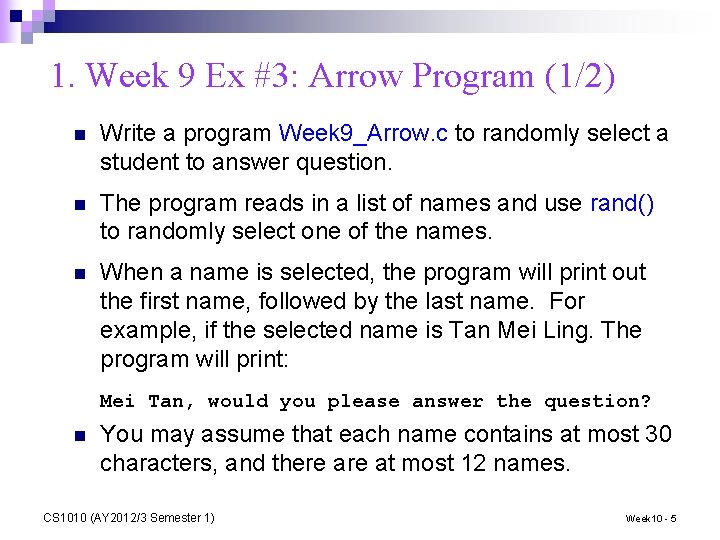1. Week 9 Ex #3: Arrow Program (1/2) n Write a program Week 9_Arrow. c to randomly select a student to answer question. n The program reads in a list of names and use rand() to randomly select one of the names. n When a name is selected, the program will print out the first name, followed by the last name. For example, if the selected name is Tan Mei Ling. The program will print: Mei Tan, would you please answer the question? n You may assume that each name contains at most 30 characters, and there at most 12 names. CS 1010 (AY 2012/3 Semester 1) Week 10 - 5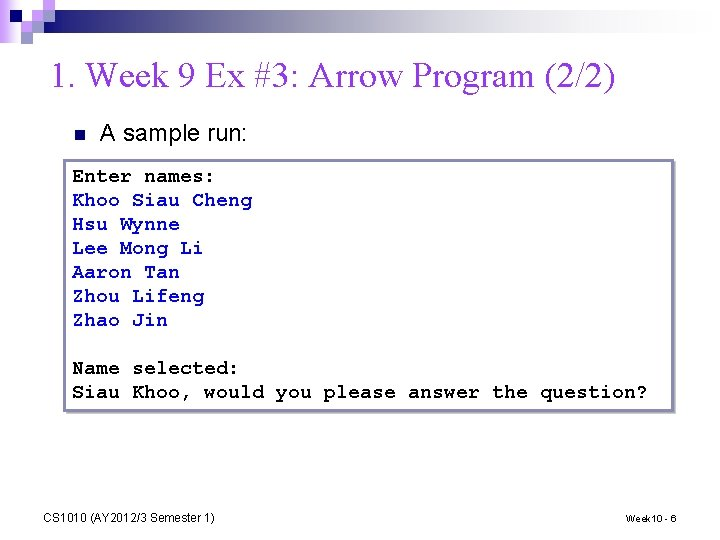1. Week 9 Ex #3: Arrow Program (2/2) n A sample run: Enter names: Khoo Siau Cheng Hsu Wynne Lee Mong Li Aaron Tan Zhou Lifeng Zhao Jin Name selected: Siau Khoo, would you please answer the question? CS 1010 (AY 2012/3 Semester 1) Week 10 - 6printf("Enter names (terminate with ctrl-d): n"); while (fgets(name. List[i], NAME_LENGTH+1, stdin) != NULL) { if (i++ >= GRP_SIZE) break; } srand(time(NULL)); index = (rand() / (double) RAND_MAX) * (i - 1); //Find the last and first name strcpy(lastname, strtok(name. List[index], " ")); strcpy(firstname, strtok(NULL, " ")); printf("Name selected: n"); printf("%s %s, would you please answer the question? n“, firstname, lastname); CS 1010 (AY 2012/3 Semester 1) Week 10 - 72. Overall Introduction n n You have accumulated quite a bit of basic programming experience by now. Today, we will study some simple yet useful classical algorithms which find their place in many CS applications ¨ ¨ ¨ Searching for some data amid very large collection of data Sorting very large collection of data according to some order How large is the collection of data that we are considering? n We will begin with an algorithm (idea), and show the algorithm is transformed into a C program (implementation). n This brings back (reminds you) our very first lecture: the importance of beginning with an algorithm. CS 1010 (AY 2012/3 Semester 1) Week 10 - 83. Introduction to Searching (1/2) n Searching is a common task that we carry out without much thought everyday. ¨ ¨ n Searching for a location in a map Searching for the contact of a particular person Searching for a nice picture for your project report Searching for a research paper required in your course. In this lecture, you will learn how to search for an item (sometimes called a search key) in an array. CS 1010 (AY 2012/3 Semester 1) Week 10 - 9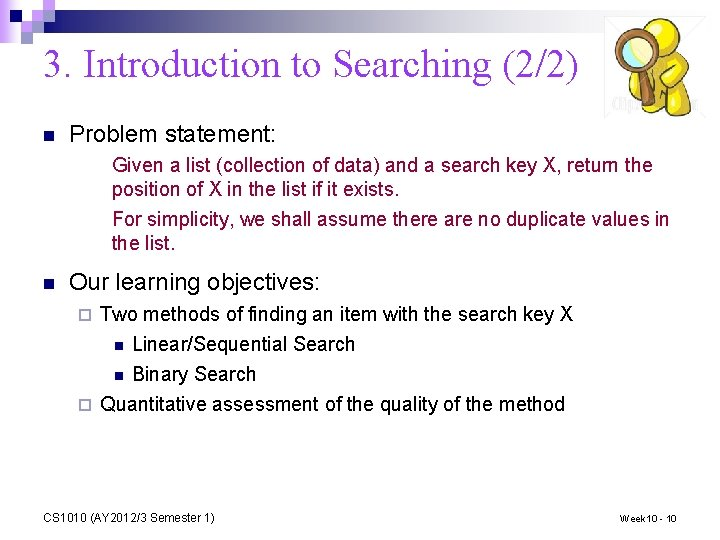3. Introduction to Searching (2/2) n Problem statement: Given a list (collection of data) and a search key X, return the position of X in the list if it exists. For simplicity, we shall assume there are no duplicate values in the list. n Our learning objectives: ¨ Two methods of finding an item with the search key X n Linear/Sequential Search Binary Search ¨ Quantitative assessment of the quality of the method n CS 1010 (AY 2012/3 Semester 1) Week 10 - 10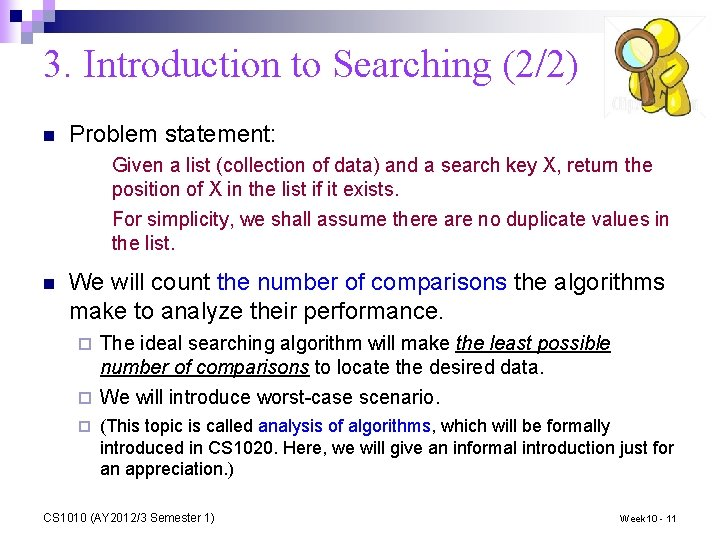3. Introduction to Searching (2/2) n Problem statement: Given a list (collection of data) and a search key X, return the position of X in the list if it exists. For simplicity, we shall assume there are no duplicate values in the list. n We will count the number of comparisons the algorithms make to analyze their performance. The ideal searching algorithm will make the least possible number of comparisons to locate the desired data. ¨ We will introduce worst-case scenario. ¨ ¨ (This topic is called analysis of algorithms, which will be formally introduced in CS 1020. Here, we will give an informal introduction just for an appreciation. ) CS 1010 (AY 2012/3 Semester 1) Week 10 - 114. Linear Search n n n Also known as Sequential Search Idea: Search the list from one end to the other end in linear progression. Example: Search for 24 Algorithm // Search for key in list A with n items linear_search(A, n, key) { for i = 0 to n-1 if Ai is key then report i } n in this list 87 12 51 9 24 63 no yes! no no no Question: What to report if key is not found? n Aim for a clean design CS 1010 (AY 2012/3 Semester 1) Week 10 - 12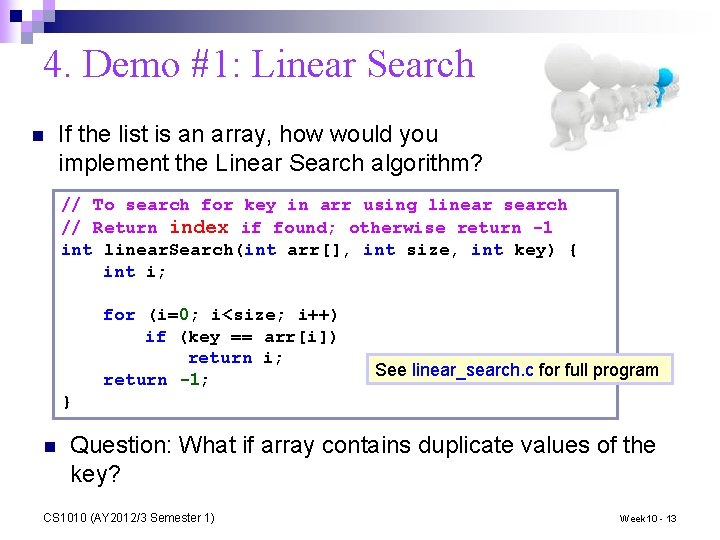4. Demo #1: Linear Search n If the list is an array, how would you implement the Linear Search algorithm? // To search for key in arr using linear search // Return index if found; otherwise return -1 int linear. Search(int arr[], int size, int key) { int i; for (i=0; i<size; i++) if (key == arr[i]) return i; return -1; See linear_search. c for full program } n Question: What if array contains duplicate values of the key? CS 1010 (AY 2012/3 Semester 1) Week 10 - 134. Linear Search: Performance Analysis n We use the number of comparisons here as a rough measurement. n Analysis is usually done for best case, average case, and worst case. We will focus on the worst case. n For an array with n elements, in the worst case, What is the number of comparisons in our linear search algorithm? n comparisons ¨ Under what circumstances do we encounter the worst case? ¨ (a) Not found (b) Found at last element CS 1010 (AY 2012/3 Semester 1) Week 10 - 14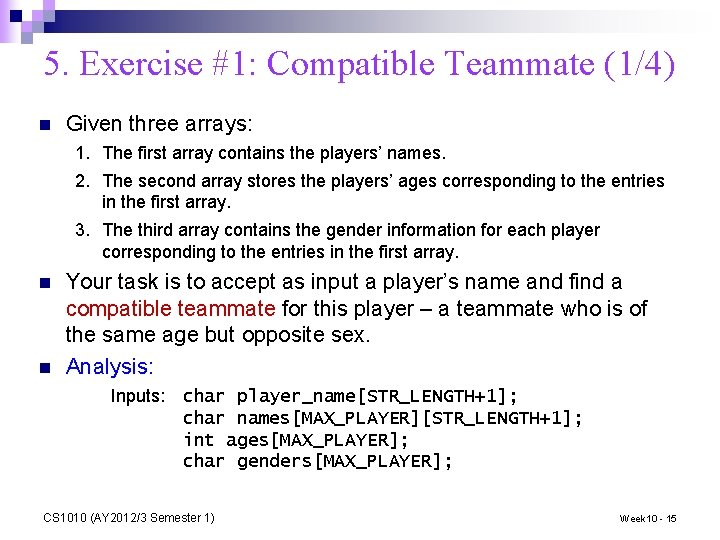5. Exercise #1: Compatible Teammate (1/4) n Given three arrays: 1. The first array contains the players’ names. 2. The second array stores the players’ ages corresponding to the entries in the first array. 3. The third array contains the gender information for each player corresponding to the entries in the first array. n n Your task is to accept as input a player’s name and find a compatible teammate for this player – a teammate who is of the same age but opposite sex. Analysis: Inputs: char player_name[STR_LENGTH+1]; char names[MAX_PLAYER][STR_LENGTH+1]; int ages[MAX_PLAYER]; char genders[MAX_PLAYER]; CS 1010 (AY 2012/3 Semester 1) Week 10 - 15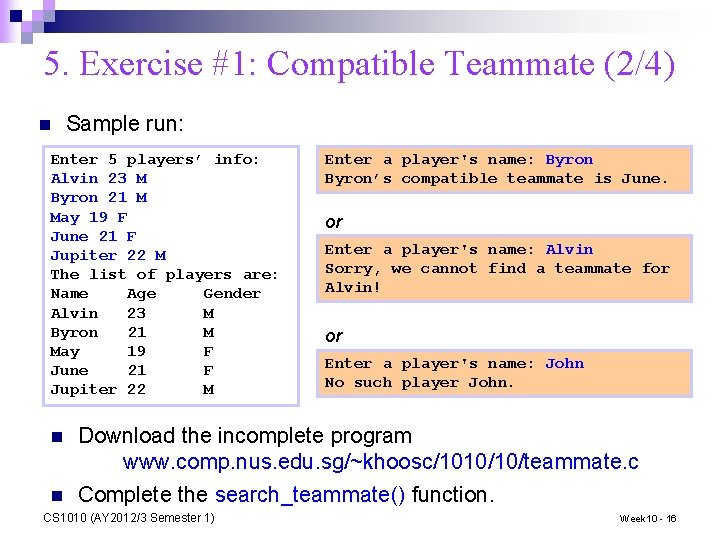5. Exercise #1: Compatible Teammate (2/4) n Sample run: Enter 5 players’ info: Alvin 23 M Byron 21 M May 19 F June 21 F Jupiter 22 M The list of players are: Name Age Gender Alvin 23 M Byron 21 M May 19 F June 21 F Jupiter 22 M n n Enter a player's name: Byron’s compatible teammate is June. or Enter a player's name: Alvin Sorry, we cannot find a teammate for Alvin! or Enter a player's name: John No such player John. Download the incomplete program www. comp. nus. edu. sg/~khoosc/1010/10/teammate. c Complete the search_teammate() function. CS 1010 (AY 2012/3 Semester 1) Week 10 - 165. Exercise #1: Compatible Teammate (3/4) int main(void) { char names[MAX_PLAYER][STR_LENGTH+ 1]; int ages[MAX_PLAYER]; char genders[MAX_PLAYER]; char player_name[STR_LENGTH+1]; int i, result; Week 10_Team. Mate. c printf("Enter %d players' info: n", MAX_PLAYER); for (i=0; i<MAX_PLAYER; i++) scanf("%s %d %c", names[i], &ages[i], &genders[i]); printf("The list of players are: n"); printf("Namet. Aget. Gendern"); for (i=0; i<MAX_PLAYER; i++) printf("%st%dt%cn", names[i], ages[i], genders[i]); printf("Enter a player's name: " ); scanf("%s", player_name); result = search_teammate(names, ages, genders, MAX_PLAYER, player_name); if (result == -2) printf("No such player %s. n", player_name); else if (result == -1) printf("Sorry, we cannot find a teammate for %s!n", player_name); else printf("%s's compatible teammate is %s. n", player_name, names[result]); return 0; } CS 1010 (AY 2012/3 Semester 1) Week 10 - 175. Exercise #1: Compatible Teammate (4/4) // Search for a player's compatible teammate // Return index in array if compatible teammate found, or // -1 if compatible teammate not found, or // -2 if player not found int search_teammate(char names[][STR_LENGTH+1], int ages[], char genders[], int size, char player_name[]) { int i, player_index = -1; // First, check that the player_name appears in the names array for (i=0; i<size; i++) { if (strcmp(player_name, names[i]) == 0) player_index = i; } if (player_index == -1) return -2; // no such student in array for (i=0; i<size; i++) { if ((ages[i] == ages[player_index]) && (genders[i] != genders[player_index])) return i; } return -1; // cannot find compatible teammate } CS 1010 (AY 2012/3 Semester 1) Week 10 - 18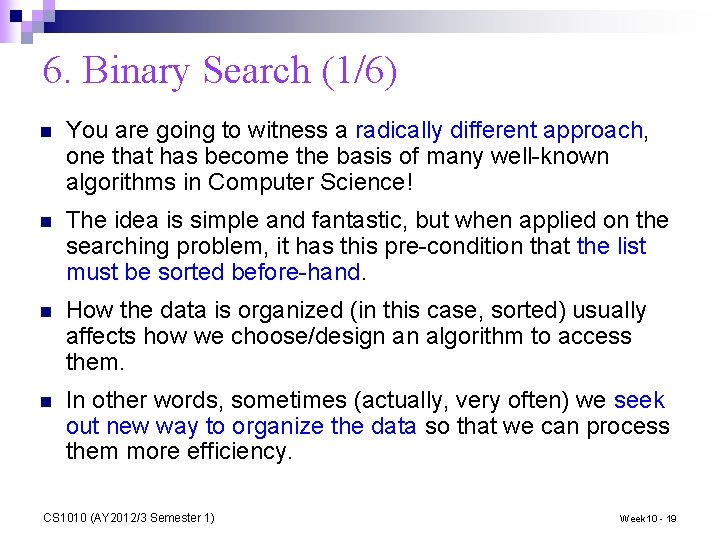6. Binary Search (1/6) n You are going to witness a radically different approach, one that has become the basis of many well-known algorithms in Computer Science! n The idea is simple and fantastic, but when applied on the searching problem, it has this pre-condition that the list must be sorted before-hand. n How the data is organized (in this case, sorted) usually affects how we choose/design an algorithm to access them. n In other words, sometimes (actually, very often) we seek out new way to organize the data so that we can process them more efficiency. CS 1010 (AY 2012/3 Semester 1) Week 10 - 19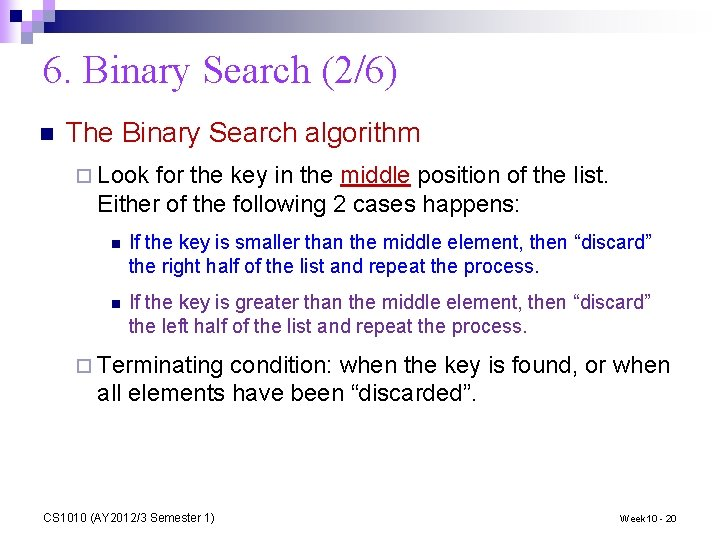6. Binary Search (2/6) n The Binary Search algorithm ¨ Look for the key in the middle position of the list. Either of the following 2 cases happens: n If the key is smaller than the middle element, then “discard” the right half of the list and repeat the process. n If the key is greater than the middle element, then “discard” the left half of the list and repeat the process. ¨ Terminating condition: when the key is found, or when all elements have been “discarded”. CS 1010 (AY 2012/3 Semester 1) Week 10 - 20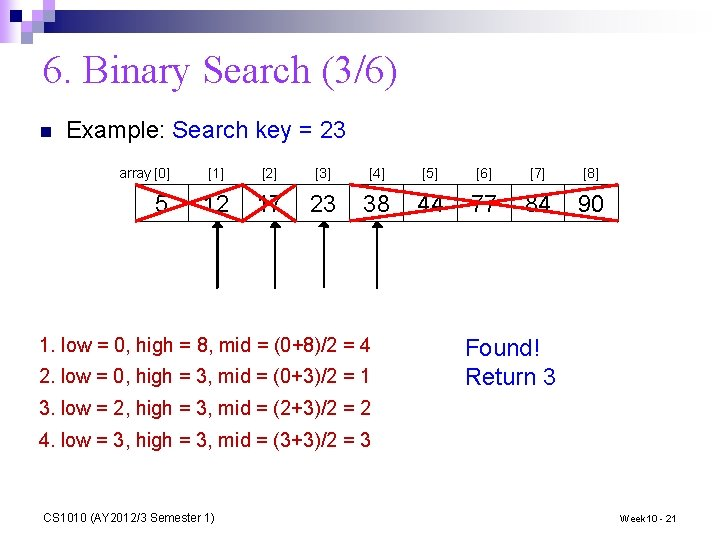6. Binary Search (3/6) n Example: Search key = 23 array  5         12 17 23 38 44 77 84 90 1. low = 0, high = 8, mid = (0+8)/2 = 4 2. low = 0, high = 3, mid = (0+3)/2 = 1 Found! Return 3 3. low = 2, high = 3, mid = (2+3)/2 = 2 4. low = 3, high = 3, mid = (3+3)/2 = 3 CS 1010 (AY 2012/3 Semester 1) Week 10 - 216. Binary Search (4/6) n In binary search, each step eliminates the problem size (array size) by half. ¨ n This is a simple yet powerful strategy, of halving the solution space in each step Lab 3 Ex 2 Bisection Method ¨ The problem size gets reduced to 1 very quickly! (see next slide) Which lab exercise employs similar strategy? n Such strategy, a special case of divide-and-conquer paradigm, can be naturally implemented using recursion (a topic we will cover next week) n But for today, we will stick to iteration (loop) CS 1010 (AY 2012/3 Semester 1) Week 10 - 22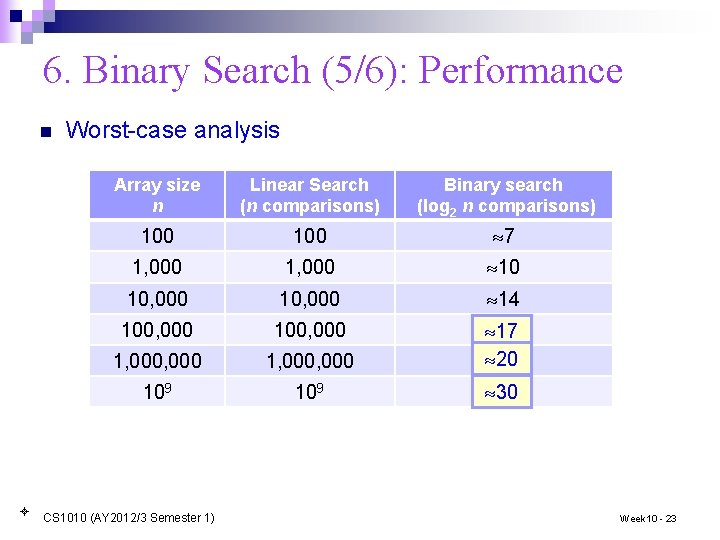6. Binary Search (5/6): Performance n Worst-case analysis Array size n Linear Search (n comparisons) Binary search (log 2 n comparisons) 100 7 1, 000 10 10, 000 14 100, 000 1, 000, 000 ? 17 20 ? 109 30 ? CS 1010 (AY 2012/3 Semester 1) Week 10 - 236. Binary Search (6/6): Code binary_search. c // To search for key in sorted arr using binary search // Return index if found; otherwise return -1 int binary. Search(int arr[], int size, int key) { int low = 0, high = size – 1, mid = (low + high)/2; while ((low <= high) && (arr[mid] != key)) { if (key < arr[mid]) high = mid - 1; else low = mid + 1; mid = (low + high)/2; } if (low > high) return -1; else return mid; } CS 1010 (AY 2012/3 Semester 1) Week 10 - 24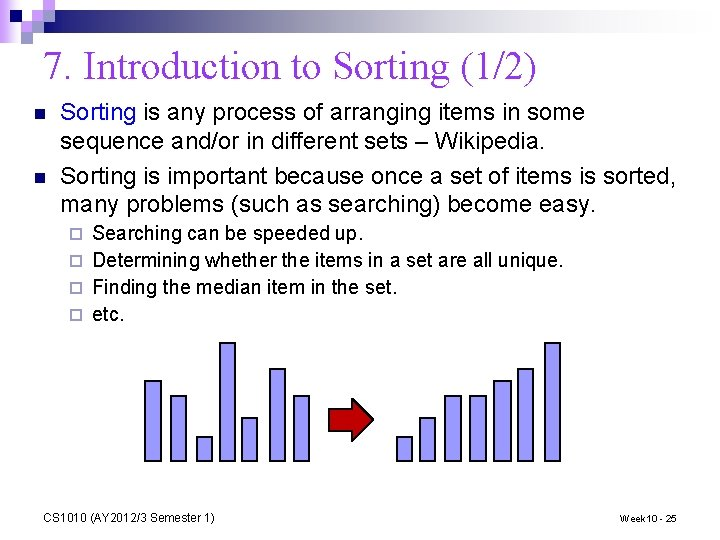7. Introduction to Sorting (1/2) n n Sorting is any process of arranging items in some sequence and/or in different sets – Wikipedia. Sorting is important because once a set of items is sorted, many problems (such as searching) become easy. Searching can be speeded up. ¨ Determining whether the items in a set are all unique. ¨ Finding the median item in the set. ¨ etc. ¨ CS 1010 (AY 2012/3 Semester 1) Week 10 - 25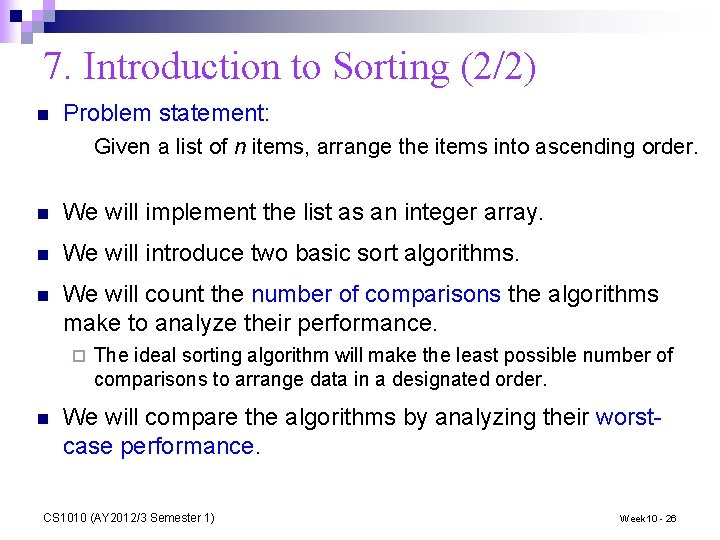7. Introduction to Sorting (2/2) n Problem statement: Given a list of n items, arrange the items into ascending order. n We will implement the list as an integer array. n We will introduce two basic sort algorithms. n We will count the number of comparisons the algorithms make to analyze their performance. ¨ n The ideal sorting algorithm will make the least possible number of comparisons to arrange data in a designated order. We will compare the algorithms by analyzing their worstcase performance. CS 1010 (AY 2012/3 Semester 1) Week 10 - 26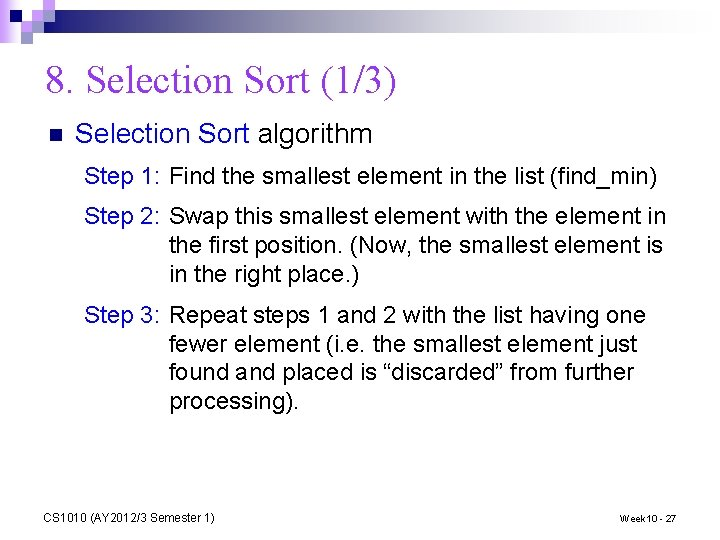8. Selection Sort (1/3) n Selection Sort algorithm Step 1: Find the smallest element in the list (find_min) Step 2: Swap this smallest element with the element in the first position. (Now, the smallest element is in the right place. ) Step 3: Repeat steps 1 and 2 with the list having one fewer element (i. e. the smallest element just found and placed is “discarded” from further processing). CS 1010 (AY 2012/3 Semester 1) Week 10 - 27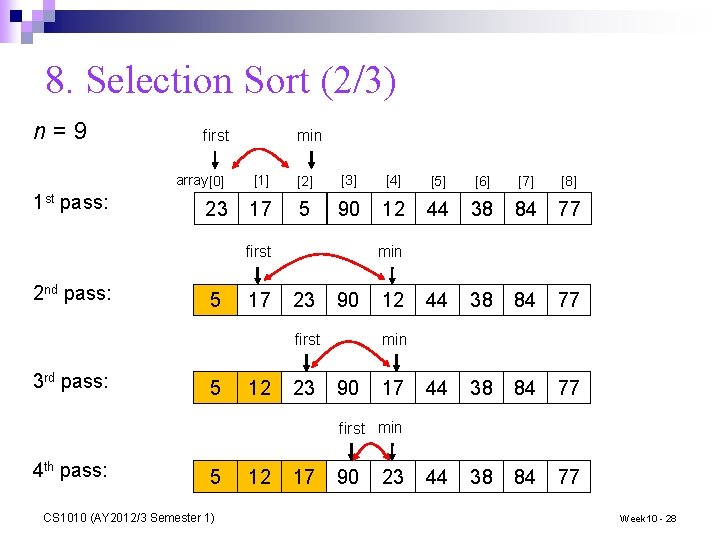8. Selection Sort (2/3) n=9 1 st pass: first min array          23 17 5 90 12 44 38 84 77 min first 2 nd pass: 5 17 23 90 min first 3 rd pass: 5 12 23 12 90 17 first min 4 th pass: 5 CS 1010 (AY 2012/3 Semester 1) 12 17 90 23 Week 10 - 28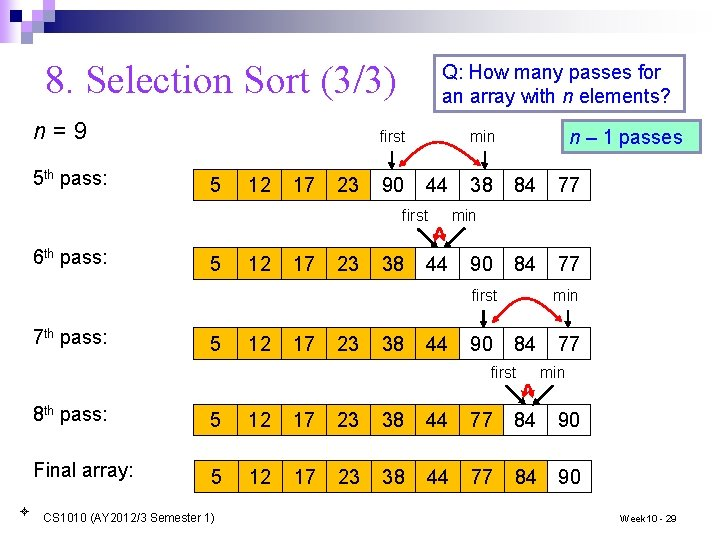8. Selection Sort (3/3) n=9 5 th pass: Q: How many passes for an array with n elements? 5 12 17 23 90 44 first 6 th pass: 5 12 17 23 38 n – 1 passes min first 44 38 84 77 min 90 min first 7 th pass: 5 12 17 23 38 44 90 84 first 77 min 8 th pass: 5 12 17 23 38 44 77 84 90 Final array: 5 12 17 23 38 44 77 84 90 CS 1010 (AY 2012/3 Semester 1) Week 10 - 298. Demo #2: Selection Sort See selection_sort. c for full program // To sort arr in increasing order void selection. Sort(int arr[], int size) { int i, start, min_index, temp; for (start = 0; start < size-1; start++) { // each iteration of the for loop is one pass // find the index of minimum element min_index = start; for (i = start+1; i < size; i++) if (arr[i] < arr[min_index]) min_index = i; // swap minimum element with element at start index temp = arr[start]; arr[start] = arr[min_index]; arr[min_index] = temp; } } CS 1010 (AY 2012/3 Semester 1) Week 10 - 308. Selection Sort: Performance n n n We choose the number of comparisons as our basis of analysis. Comparisons of array elements occur in the inner loop, where the minimum element is determined. Assuming an array with n elements. Table below shows the number of comparisons for each pass. The total number of comparisons is calculated in the formula below. Such an algorithm is call an n 2 algorithm, or quadratic algorithm, in terms of running time complexity. Pass #comparisons 1 n– 1 2 n– 2 3 n– 3 … … n– 1 1 CS 1010 (AY 2012/3 Semester 1) Week 10 - 318. Selection Sort n Selection sort is classified under exchange sort, where elements are exchanged in the process n We could search for the minimum element as described earlier, or search for the maximum element and exchange it with the last element of the working array (assuming we sort in ascending order) n The latter is described in Lesson 6. 6 in the book as an Exchange Maximum Sort CS 1010 (AY 2012/3 Semester 1) Week 10 - 329. Exercise #2: Points and Lines (1/6) n Problem: You are given a list of points on a 2 -dimensional plane, each point represented by its integer x- and y-coordinates. You are to sort the points in ascending order of their x-coordinates, and for those with the same x-coordinate, in ascending order of their y-coordinates. n Two arrays are used to store the points: array x for their xcoordinates, and array y for their y-coordinates. x[i] and y[i] refer to the point i. n You may assume that there at most 20 points and no two points are the same. n Do the sorting by calling Selection Sort only once. How do you adapt the Selection Sort code for this problem? n You are given an incomplete program Week 10_Points. c n This exercise is mounted on Code. Crunch. CS 1010 (AY 2012/3 Semester 1) Week 10 - 33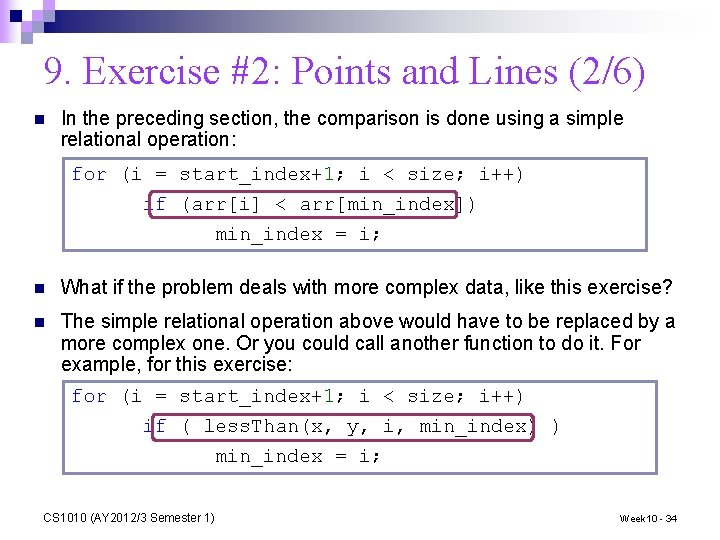9. Exercise #2: Points and Lines (2/6) n In the preceding section, the comparison is done using a simple relational operation: for (i = start_index+1; i < size; i++) if (arr[i] < arr[min_index]) min_index = i; n What if the problem deals with more complex data, like this exercise? n The simple relational operation above would have to be replaced by a more complex one. Or you could call another function to do it. For example, for this exercise: for (i = start_index+1; i < size; i++) if ( less. Than(x, y, i, min_index) ) min_index = i; CS 1010 (AY 2012/3 Semester 1) Week 10 - 349. Exercise #2: Points and Lines (3/6) n Here’s the incomplete less. Than() function: // Returns 1 if point at index p is "less than" point at index q; // otherwise returns 0. // Point at index p is "less than" point at index q if the former // has a smaller x-coordinate, or if their x-coordinates are the // same, then the former has a smaller y-coordinate. int less. Than(int x[], int y[], int p, int q) { return (x[p] < x[q]) || ( (x[p] == x[q]) && (y[p] < y[q]) ); } CS 1010 (AY 2012/3 Semester 1) Week 10 - 35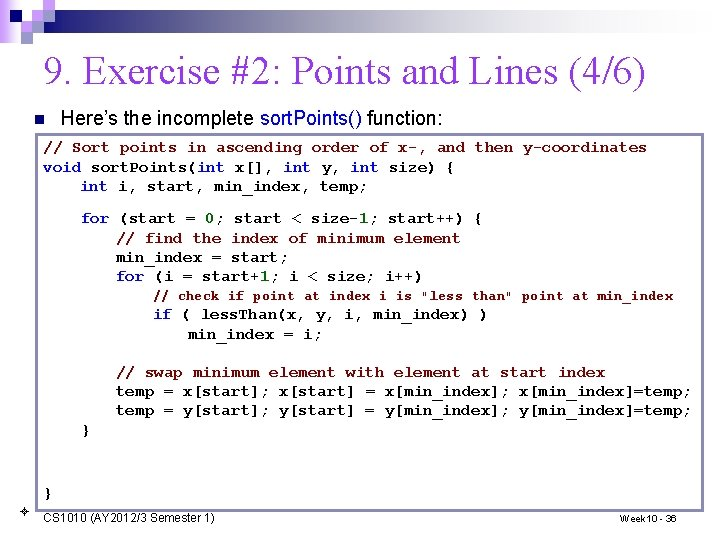9. Exercise #2: Points and Lines (4/6) n Here’s the incomplete sort. Points() function: // Sort points in ascending order of x-, and then y-coordinates void sort. Points(int x[], int y, int size) { int i, start, min_index, temp; for (start = 0; start < size-1; start++) { // find the index of minimum element min_index = start; for (i = start+1; i < size; i++) // check if point at index i is "less than" point at min_index if ( less. Than(x, y, i, min_index) ) min_index = i; // swap minimum element with element at start index temp = x[start]; x[start] = x[min_index]; x[min_index]=temp; temp = y[start]; y[start] = y[min_index]; y[min_index]=temp; } } CS 1010 (AY 2012/3 Semester 1) Week 10 - 369. Exercise #2: Points and Lines (5/6) n [Do this part after class] After sorting the points, imagine that you trace the points in their order in the sorted array. Write a function trace. Lines() to compute the sum of the lengths of those lines that are horizontal or vertical. n For example, after sorting, here are the points: (1, 2), (1, 3), (2, 1), (2, 4), (3, 2), (3, 3), (3, 4), (5, 3), (5, 6), (6, 2), (6, 5), (7, 2), (10, 4), (11, 4), (12, 2). The vertical and horizontal lines are marked in green. 6 5 4 (1, 3) 3 (1, 2) 2 1 2 3 4 5 CS 1010 (AY 2012/3 Semester 1) 6 7 8 9 10 11 12 n Sum of lengths of horizontal and vertical lines = 1 + 3 + 2 + 3 + 1 = 13 1 Week 10 - 37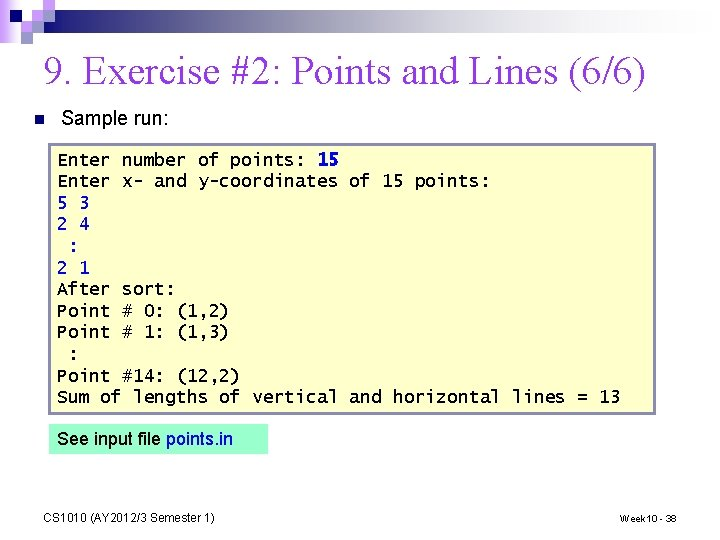9. Exercise #2: Points and Lines (6/6) n Sample run: Enter number of points: 15 Enter x- and y-coordinates of 15 points: 5 3 2 4 : 2 1 After sort: Point # 0: (1, 2) Point # 1: (1, 3) : Point #14: (12, 2) Sum of lengths of vertical and horizontal lines = 13 See input file points. in CS 1010 (AY 2012/3 Semester 1) Week 10 - 38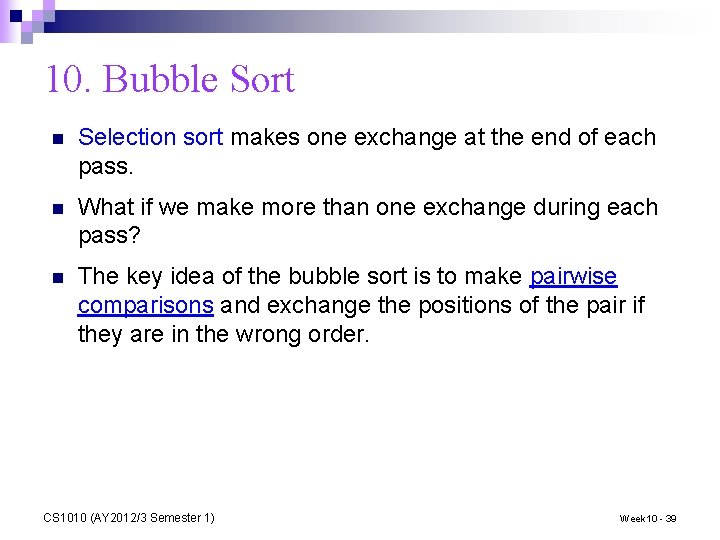10. Bubble Sort n Selection sort makes one exchange at the end of each pass. n What if we make more than one exchange during each pass? n The key idea of the bubble sort is to make pairwise comparisons and exchange the positions of the pair if they are in the wrong order. CS 1010 (AY 2012/3 Semester 1) Week 10 - 39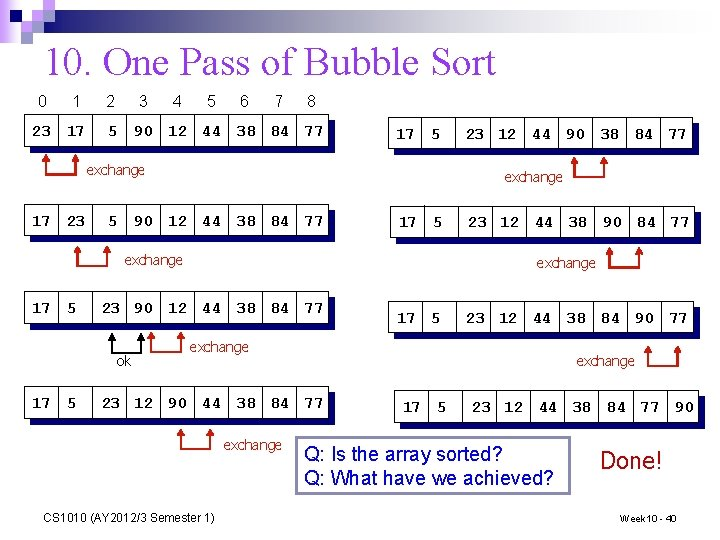10. One Pass of Bubble Sort 0 1 23 17 2 3 5 4 5 6 7 8 90 12 44 38 84 77 17 5 exchange 17 23 5 exchange 90 12 44 38 84 77 17 5 exchange 17 5 23 12 44 38 90 84 77 exchange 23 90 12 44 38 84 77 ok 23 12 44 90 38 84 77 17 5 23 12 44 38 84 90 77 exchange 23 12 90 44 38 84 77 exchange CS 1010 (AY 2012/3 Semester 1) 17 5 23 12 44 38 84 77 90 Q: Is the array sorted? Q: What have we achieved? Done! Week 10 - 40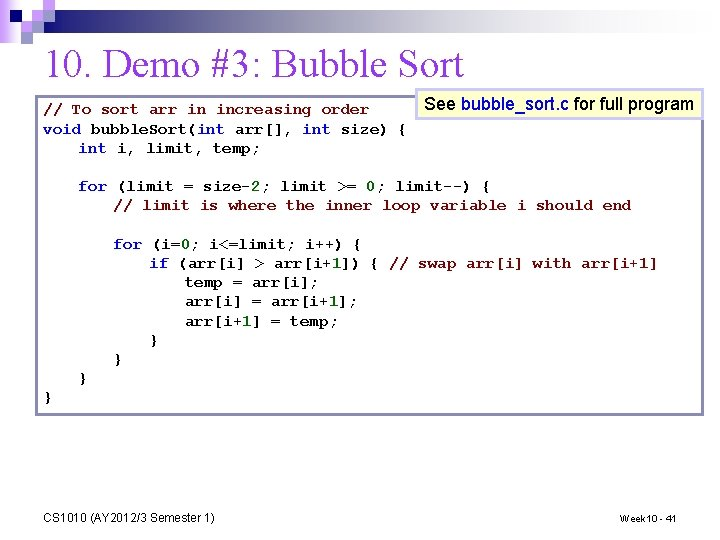10. Demo #3: Bubble Sort See bubble_sort. c for full program // To sort arr in increasing order void bubble. Sort(int arr[], int size) { int i, limit, temp; for (limit = size-2; limit >= 0; limit--) { // limit is where the inner loop variable i should end for (i=0; i<=limit; i++) { if (arr[i] > arr[i+1]) { // swap arr[i] with arr[i+1] temp = arr[i]; arr[i] = arr[i+1]; arr[i+1] = temp; } } CS 1010 (AY 2012/3 Semester 1) Week 10 - 4110. Bubble Sort Performance n Bubble sort, like selection sort, requires n – 1 passes for an array with n elements. n The comparisons occur in the inner loop. The number of comparisons in each pass is given in the table below. The total number of comparisons is calculated in the formula below. Like selection sort, bubble sort is also an n 2 algorithm, or quadratic algorithm, in terms of running time complexity. n n Pass #comparisons 1 n– 1 2 n– 2 3 n– 3 … … n– 1 1 CS 1010 (AY 2012/3 Semester 1) Week 10 - 42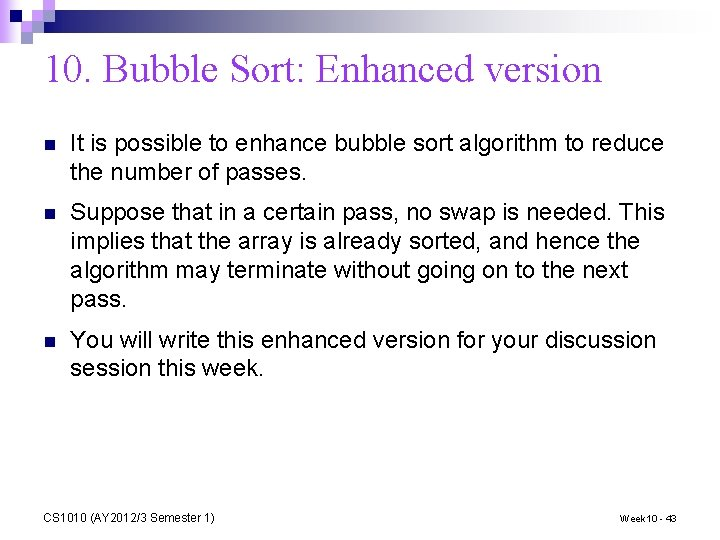10. Bubble Sort: Enhanced version n It is possible to enhance bubble sort algorithm to reduce the number of passes. n Suppose that in a certain pass, no swap is needed. This implies that the array is already sorted, and hence the algorithm may terminate without going on to the next pass. n You will write this enhanced version for your discussion session this week. CS 1010 (AY 2012/3 Semester 1) Week 10 - 43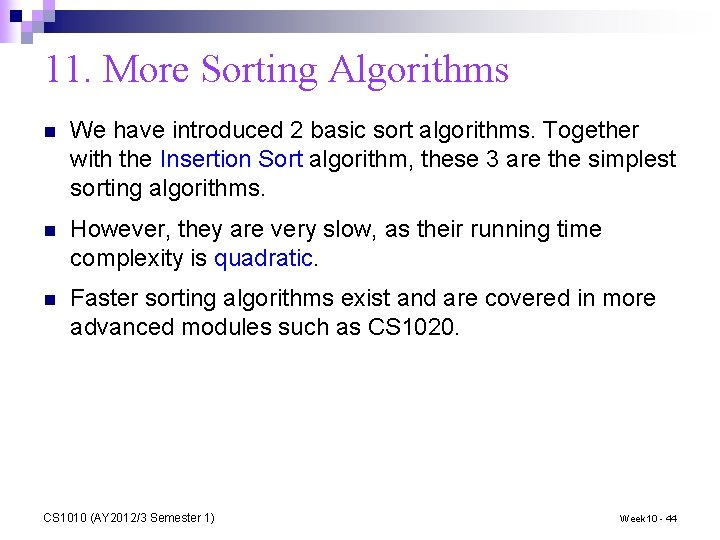11. More Sorting Algorithms n We have introduced 2 basic sort algorithms. Together with the Insertion Sort algorithm, these 3 are the simplest sorting algorithms. n However, they are very slow, as their running time complexity is quadratic. n Faster sorting algorithms exist and are covered in more advanced modules such as CS 1020. CS 1010 (AY 2012/3 Semester 1) Week 10 - 4412. Animated Sorting Algorithms n There a number of animated sorting algorithms on the Internet n Here are two sites: http: //www. sorting-algorithms. com/ ¨ http: //www. cs. ubc. ca/~harrison/Java/sorting-demo. html ¨ n There also folk dances based on sorting! ¨ Selection sort with Gypsy folk dance http: //www. youtube. com/watch? v=Ns 4 TPTC 8 whw ¨ Bubble-sort with Hungarian folk dance http: //www. youtube. com/watch? v=ly. ZQPj. UT 5 B 4 CS 1010 (AY 2012/3 Semester 1) Week 10 - 4513. Exercise #3: Module Sorting (takehome) (1/2) n Week 10_Sort. Modules. c: Given two arrays: one containing the module codes, and the other containing the number of students enrolled in the modules. Sort the modules in ascending order of student enrolment, using Selection Sort, Bubble Sort, or Insertion Sort (if you happen to know it). n You may assume that there at most 10 modules and a module code is at most 7 characters long. n This is a take-home exercise. n This exercise is mounted on Code. Crunch. CS 1010 (AY 2012/3 Semester 1) Week 10 - 46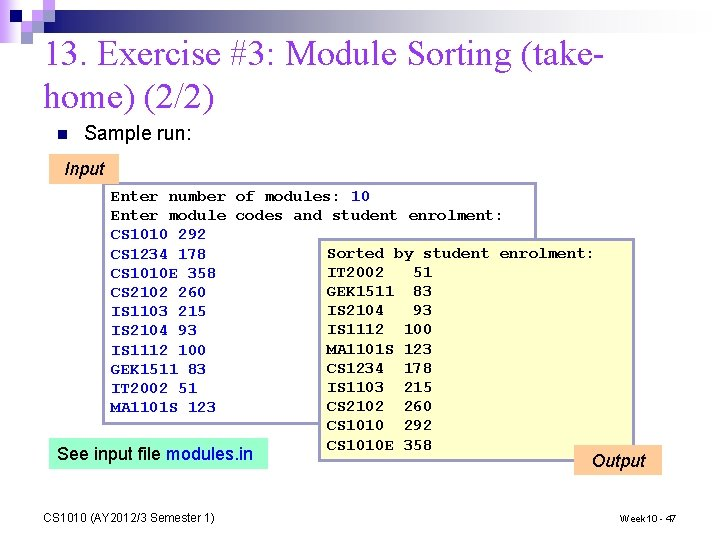13. Exercise #3: Module Sorting (takehome) (2/2) n Sample run: Input Enter number of modules: 10 Enter module codes and student enrolment: CS 1010 292 Sorted by student enrolment: CS 1234 178 IT 2002 51 CS 1010 E 358 GEK 1511 83 CS 2102 260 IS 2104 93 IS 1103 215 IS 1112 100 IS 2104 93 MA 1101 S 123 IS 1112 100 CS 1234 178 GEK 1511 83 IS 1103 215 IT 2002 51 CS 2102 260 MA 1101 S 123 CS 1010 292 CS 1010 E 358 See input file modules. in CS 1010 (AY 2012/3 Semester 1) Output Week 10 - 47Summary for Today n Searching algorithms n n n Linear (sequential) search Binary search Basic sorting algorithms n n Selection sort Bubble sort CS 1010 (AY 2012/3 Semester 1) Week 10 - 48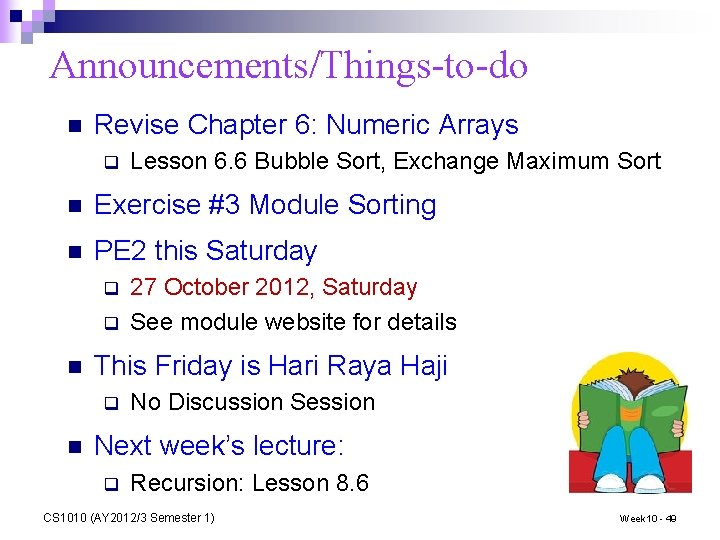Announcements/Things-to-do n Revise Chapter 6: Numeric Arrays q Lesson 6. 6 Bubble Sort, Exchange Maximum Sort n Exercise #3 Module Sorting n PE 2 this Saturday q q n This Friday is Hari Raya Haji q n 27 October 2012, Saturday See module website for details No Discussion Session Next week’s lecture: q Recursion: Lesson 8. 6 CS 1010 (AY 2012/3 Semester 1) Week 10 - 49End of File